# Selective Medical Image Encryption Using Polynomial-Based Secret Image Sharing and Chaotic Map

Selective Medical Image Encryption Using Polynomial-Based Secret Image Sharing and Chaotic Map
Control and Systems Eng. Dept., University of Technology-Iraq, Baghdad 10066, Iraq

Corresponding Author Email:
Page:
357-369
|
DOI:
https://doi.org/10.18280/ijsse.120310
27 April 2022
|
Accepted:
10 June 2022
|
Published:
30 June 2022
| Citation

OPEN ACCESS

Abstract:

The progressive development in telecommunication and networking technologies has led to the increased popularity of telemedicine usage, which involves the storage and transfer of medical images and related information. Recently, trust and privacy in the telemedicine system have attracted many researchers to investigate these topics. In medical image applications, selective image encryption plays an important role as it reduces computational cost and time. Therefore, a safe and efficient selective image encryption algorithm is designed in this work. In particular, the predetermined region of the original image data is encrypted to reduce the encryption/decryption time and the computational complexity of processing the huge image data. The image processing techniques are used to divide the image into a region of interest (ROI) and a region of non-interest (RONI), and then the more important component of the ROI is encrypted using a polynomial-based secret image sharing (SIS) and a chaotic map system. These techniques produce a test image cipher that has good confusion and diffusion properties. The experimental result shows that the Polynomial-based SIS and the chaotic image encryption are effectively performed for diffusion and confusion, which are crucial for concealment. According to the security research findings, sensitive encryption and decryption systems are extremely reliant on any improvement in the key. The encryption solution is sufficiently broad to withstand brute force attacks. Thus, protection may become an issue during the transmission of medical images via a network.

Keywords:

selective encryption, secret image sharing, Thien and Lin’s secret SIS, medical image, chaotic map, encryption

1. Introduction

Additional computational efforts are required for redundancies, the storage and exchange of high-definition images, and important documents correlation at a greater rate of transmission, as does the encryption of such images. This will have a significant impact on the balancing of synchronization and security for real-time applications. Furthermore, data selective encryption is preferable compared to complete image encrypting, when a high designation, short memory, and lower power are resource constraints. In selective encryption, the computations’ time decreases dramatically. In this regard, the image of the document is composed of segments that are correlated and uncorrelated. Encrypting certain relative sections is preferable compared to the whole image encryption. To this end, the fundamental concept of selective encryption is firstly identified, and then the important pixels or regions of pixels are encrypted. Partial encryption also covers the encryption of data at varying levels of security to meet the needs of the end user. To meet the requirements mentioned above in terms of security and computational time for real-time applications, a large section should be dynamically or statistically selected from the whole image for purposes of partial encryption. As a result, by decreasing the computation size, partial encryption improves the efficacy of encryption .

Due to the nature of the image file structure, it is difficult to employ the image full encryption to encrypt only a section of an image. As a result, consumers are unable to recognize an encrypted image since all information data have to be encrypted. Furthermore, the users' privacy data could violate the user if released. In such an image, the face and a certain part of the body can be regarded as private data that can be used to identify and distinguish an individual. In this regard, the proportion of the encipher image can be amplified by padding using a block cipher, which is commonly used to encrypt text information for image encryption. Principally, the storage space may be squandered when the quantity of data in an image rises owing to padding in each frame . Full encryption and partial encryption are the two methods used for protecting an image from leakage and eavesdropping. The entire image encryption is carried out when it is essential to execute encryption efficiently and rapidly [3, 4]. However, selective encryption is the process of encrypting the selected part from the main image. To this end, the selective cipher process decreases the cipher and decrypting time and it also improves the image's resilience , keeping in mind that the complete encryption scheme necessitates additional calculations, slower calculation speeds, and increased equipment needs.

Several picture encryption techniques based on chaotic maps have been presented in recent years. Specifically, the permutation-diffusion technique is used in the majority of these techniques. Chaotic systems have an imperative possession, such as transitivity of topology, the sensitive reliance on preliminary situations and parameters of the system, and finally, the density of the set of all periodic points. The majority of features belong to cryptographic requirements such as diffusion and mixing. As a result, chaotic cryptosystems have a broader range of practical applications .

Choosing the region of interest cipher schemes is just as important as the encryption system. In this context, ROI encryption techniques can be classified into two groups based on the mechanism used to determine the privacy region: automatic selection encryption and manual selection encryption. More specifically, the ROI manual selection means that the user can determine and select a certain privacy area, and then the user can encrypt the desired area [7, 8]. However, the ROI manual selection method is regarded as an imprecise and time-consuming method. As a result, many research works have been carried out to suggest several automatic selection organizations.

The purpose of this work is to demonstrate how an ROI selection method based on hybrid image processing techniques is used to attain an automatic selection and a precise ROI selection in medical images. Then, for medical image ROI, a selective image encryption/ decryption algorithm is proposed based on employing polynomial-based SIS and chaotic map.

2. Related Work

Unfortunately, the traditional methods that are usually used to cipher the whole image are not very effective for encryption in huge data because the encryption processing time is fairly essential, specifically in emergency cases. To this end, several approaches have been proposed to lower the whole processing time. For instance, Goel and Chaudhari  proposed a partial encryption method based on uncomplicated mathematics that takes the average of pixel values in a block of an image. Then, the aim pixels to be encrypted were estimated by finding the median percentage deviation. In addition, Belazi et al.  presented two contributions for encryption by a chaos-based S-box which was constructed based on the chaotic Chebyshev map, the linear fractional transform, and a partial image, which was based on a permutation-substitution-diffusion network and numerous chaotic maps in the LWT transform domain of the images. However, the drawback of this method is that it is a complex and long-lasting process because there are many analyses to be done in a single technique.

In another work, Som et al.  proposed a chaotic system-based partial image encryption that was evaluated in terms of a set of evaluation criteria. The suggested approach decomposes the raw image into bit planes. Following decomposition, only the significant bit planes are chosen for encryption. Particularly, encryption was accomplished using a chaotic system to generate a pseudorandom number sequence. Nonetheless, the primary drawback of this work is that there is a great deal of confusion during the procedure.

Moreover, Ismail et al.  presented the generalization of the Double-Humped logistic map by adding an extra parameter. In particular, an image encryption algorithm was presented and established on pseudo-random sequence generation using the suggested generalized DH map. Subsequently, the dynamic performance of the generalized map was evaluated, embracing the study of the fixed points, stability ranges, and the complete bifurcation diagram. In addition, Sankaradass et al.  suggested gray-scale encryption with a chaotic technique as a new encryption technology based on the region of interest to extract the image's ROI. In this method, the Sobel edge detection techniques were utilized, in which the Sobel operator divides the suspected region from the rest of the image based on the edges present on different blocks. Additionally, the ROI data was encrypted using the Lorenz system, while the remaining regions were encrypted using a sine map. The entire image was then shuffled using the Lorenz algorithm with the most recent initial conditions, resulting in the encrypted final image. Darwish  suggested a selective image encryption method that ratifies a 3D chaotic map to de-correlate relationships between pixels in combination with an adaptive thresholding system. The proposed system was based on engaging encryption on the most noteworthy part of the image after transformation. Furthermore, Zhou et al.  presented a study on predominantly bargain (ROI) and also protected it by keeping its confidential information. Interestingly, the transfer domain encryption was also recovered without any information losses during the whole process. For medical image applications, a method of new lossless image encryption based on game theory with optimized ROI parameters and hidden ROI positions was presented. On the other hand, Khashan and AlShaikh  proposed a simple yet effective method for encrypting the edge maps of medical images. In this method, an edge detection approach was used to retrieve the edge map. Then, using a chaotic map, a vast key space was generated. In particular, this solution utilizes a one-time pad mechanism to encrypt the significant recognized image blocks, but has the problem of making some data more accessible to breaches, as not every edge device comes equipped with the same built-in authentication and security capabilities.

Cun et al.  stated that the method of encryption of a partial image depends on coding a chaotic map of dynamic DNA. Firstly, a one-dimensional chaotic map was constructed with a greater Lyapunov exponent and a more effective dynamic performance. Then, a local graph structure algorithm has been improved to better select the image area. The authors then used the constructed chaotic map to generate a pseudo-random sequence, dynamically encoding and manipulating selected DNA regions, and finally producing a fully scrambled image. In addition, Wen et al.  proposed an image cryptosystem using a diffusion-permutation-diffusion structure and they introduced a plaintext association mechanism to enhance the security. The main contribution of this work was to provide a security-enhanced image cryptosystem with low computational complexity. However, this paper still lacks consideration in terms of key management. A high chance of error in key generation is still the main problem. Recently, Pandurangi Ramacharya et al.  proposed a method for encrypting partial images quickly using fuzzy logic and chaotic mapping. The suggested technology provides an automatic picture encryption method as an alternative to human color image encryption. To pick the area to encrypt, fuzzy rules based on color details were applied. However, the methods based on fuzzy logic approaches showed poor effectiveness for illuminated and darkened images, since they are not able to significantly alter the histogram shift to correct distortion of brightness.

3. Secret Image Sharing

Image sharing is very important in the field of digital image security. In this regard, the main concept of image sharing is to divide secret data into parts and distribute them to others. Certain people can restore the entire secret. In general, image sharing can be defined as the process of splitting a secret image into n sub images each of which has some feasibilities but it does not transfer other information. To facilitate image sharing and encryption, the gray values of an image are divided using an indeterminate equation. Secret images can be reconstructed from complete knowledge using the r sub-image, where r≤n .

3.1 Shamir’s secret sharing scheme

Shamir developed the concept of a secret sharing approach based on (k, n) thresholds (k≤n). This technique enables the construction of a polynomial function of order (k-1) as,

f(x) =d0+d1x+d2x2+..+dk−1xk−1(modp)             (1)

in which the d0 value is the secret and p is a prime number. The secret has pairs of values (xi, yi) in which yi=f(xi), 1≤in, and the points are 0<x1<x2...xn≤p-1. The polynomial function f(x) is destroyed. Each shareholder has a pair of values (xi, yi), so that no single shareholder knows the secret value d0. In fact, a group of secret shares with K-1 or less cannot discover secrecy d0. However, if more than k secret shares are available, then at least k linear equations yi = f(xi) for the unknown di’s can be set. The only explanation to these equations demonstrates that the secret value d0 can be simply achieved. Shamir’s secret sharing (SSS) is regarded as a perfect secret sharing (PSS) scheme because even knowledge of the (k − 1) linear equations does not reveal information about the secrets .

3.2 Thien and Lin’s secret sharing scheme

Thien and Lin enhanced Shamir's concept in 2002 by proposing an SIS system constructed on the (r, n)-threshold technique. In this method, each generated shadow image will be 1 / r the size of the secret image. Their method evaluates the arithmetic operation of the prime Galois field GF (251) so that preprocessing is required to truncate pixel values more than 250. Suppose that there is a secret image O with m pixels, to encode O to n shadow images, the sharing steps of the Thien–Lin (r, n) SIS scheme, where 2 ≤r ≤n, are given below as S1, S2, S4,..., Sn [20-22].

Step 1. Firstly, pixel values in O that are more than 250 (251 to 255) are truncated to 250, where O' represents the image after truncation.

Step 2. Using a secret key, generate a permutation sequence in order to permute the pixels in O'; then the permutated image is symbolized by Q.

Step 3. Fix the value of the existing processing section j to one.

Step 4. Consecutively get r non-processed pixels, a0, a1, a2,…, ar–1, of Q to form a segment j, and produce a polynomial of degree r – 1, as shown below:

$f_{i}(x)=\left(a_{0}+a_{1} x+a_{2} x^{2}+\cdots+a_{r-1} x^{r-1}\right)$             (2)

Step 5. Create n pixels:

$f(1), f(2), \ldots ; f(n) n$            (3)

and assign them progressively to the n shadow images S1, S2, S4,...,Sn.

Step 6. Increase the value of j by one.

Step 7. Repeat Steps 4–6 until all pixels in Q have been processed

This approach uses most of the coefficients in Eq. (2) in order to share the secret pixels. Therefore, the shadow images’ size will be 1/r. This is a lossy secret image sharing method in which there is no prediction to thoroughly recover the first image O even when the visual quality is good. In order not to lose the generality, we assume the r shares are S1, S2, S4,…, Sr, and the following steps can be used to reveal the secret image O’ using any r (2 ≤ rn) of the shadow images for the sharing phase of the Thien–Lin (r, n)-SIS scheme.

Step 1. Fix the current number j to one.

Step 2. From each of the r shadow images, extract one unprocessed pixel.

Step 3. Using these r pixels, fj(1), fj(2), fj(4),..., fj(r), and Lagrange's interpolation, calculate the equation for the coefficients a0, a1, a2,..., ar–1 (2), representing the jth section's corresponding r pixel values.

Step 4. Increase the value of j by one.

Step 5. Repeat Steps 2–4 until all pixels in the shadow pictures S1, S2, S4,..., Sr have been processed.

Step 6. Use the inverse-permutation process to the permuted image Q in order to recover the secret image to O’.

Figure 1 represents a sample of (2, 4) image secret share construction process in which n = 4 and k = 2, where first order polynomial functions are produced as the following equation:

$S x(i, j)=(110+112 x)(\bmod 251)$          (4)

The first two pixel value are (110 and 112) in the Lena image .

## 1.png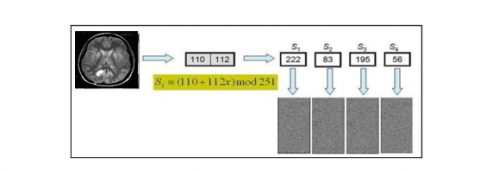Figure 1. Thien & Lin's secret sharing scheme 

As demonstrated by its simple mathematical formulations, the quadratic map exhibits extremely complicated dynamic features , which correspond to the non-asymptotic behavior of reiterations at n→+∞. Additionally, the characteristics may vary significantly depending on the value of parameter a. This is related to the fact that for big n, due to its highly polynomial degree, it is extremely dependent on x and in a complex fashion. In this regard, quadratic mapping can be utilized to comprehensively capture these dynamics. Consider the following quadratic equation:

$X_{n+1}=a-x_{n}^{2} \quad$ for $0<a<2$          (5)

where, the fixed points are $x_{n}$. The solution is likely to vary around one of the fixed points if the map is reiterated. It either attracts or repels a fixed point. Specifically, there is opposition and attraction in the instance of a quadratic chaotic map. If a fixed point exhibits attraction, it will remain stable. Repulsion indicates that the fixed point is unstable. To have a comprehensive understanding, it is necessary to identify and study the fixed points. It is worth noticing that linearity may be applied in this case as well. In this suggested work, picture pixels are permuted using the quadratic chaotic map , as depicted in Figure 2.

The quadratic map, as illustrated in Figure 3, might yield unnecessary sequences. As a result, the chaotic sequence formed is extremely dependent on the original situation. A small change in the initial condition results in an entirely new chaotic sequence.

## 2.png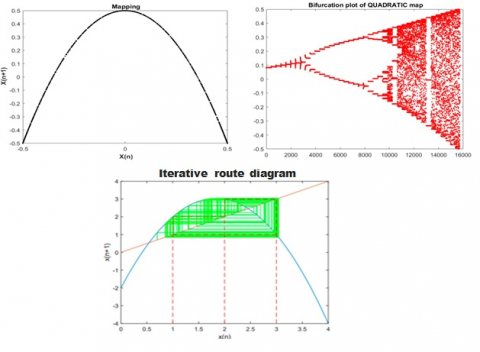Figure 2. Quadratic chaotic map

## 3.png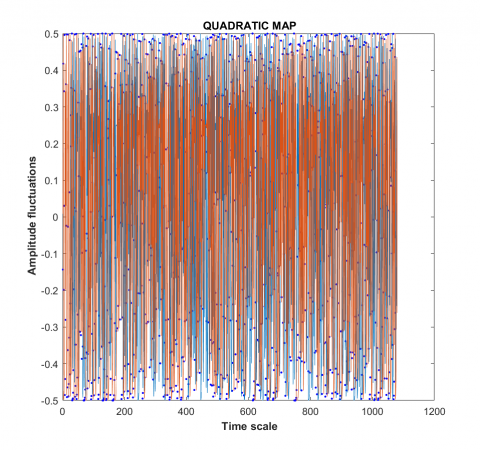Figure 3. Sensitivity to initial conditions of two quadratic chaotic signals

## 4.png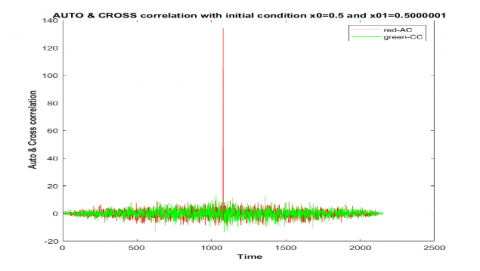Figure 4. The auto correlation performance of the quadratic chaos generator

Figure 4 shows the behavior of the cross and auto correlation of the quadratic chaos generator with the initial state’s different values. The properties of the quadratic of an auto- and cross-correlation are similar to the characteristics of a random value to the white noise, although there is a little difference. Due to the significant behavior of autocorrelation properties, the quadratic chaos generator is used in security applications.

4. Proposed System

In the proposed system, computationally efficient techniques are adopted for maintaining the storage space and achieving confidential transmission of medical image data. More precisely, the partial encryption technique based on the chaotic system and polynomial is proposed. The encryption is applied on the ROI of the medical image (i.e., tumor) for confidentiality. The proposed system’s block diagram is shown in Figure 5. In particular, automated brain tumor detection of the MRI image based on hybrid image processing techniques is performed on the images to localize the tumor regions of interest .

## 5.png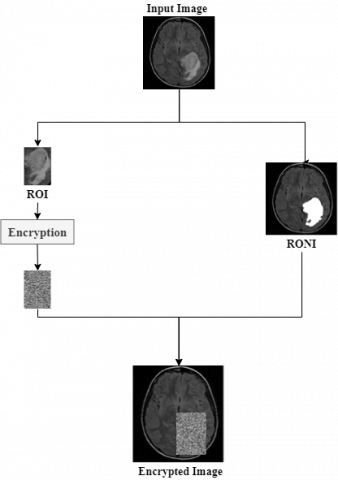Figure 5. A block diagram of the presented system

4.1 Design philosophy of the proposed encryption

The following points are the building blocks of the proposed encryption algorithm design:

• The presented system has a block size of 256 bits, which supports the largest bit size and is practically unbreakable by brute force based on the current computing power.
• One of the proposed encryption's important features is that it employs a chaining-like process that causes the decryption of a ciphertext block to depend on the preceding ciphertext blocks. As a result, the entire validity of preceding blocks is contained in the previous, adjacent ciphertext block. In this regard, a single bit error in a ciphertext block affects the decryption of all subsequent blocks.
• The proposed system uses a large key space, which has to be larger than 2100 to overcome certain attacks like brute force. In other words, the large key space is essential to overcome the issue of a comprehensive search for a key.
• The proposed system uses more complex mathematical tools like the polynomial and chaotic map to raise the security and improve the encryption system sensitivity.

4.2 Key generation scheme

The key generation process depends on the hash code. Particularly, the hash function extracts the features from the region of interest based on SHA-512. It can produce a 512-bits hash value . As SHA-512 is permanent, it can resist diverse types of attacks, such as plaintext attacks. The main generation steps of the encryption scheme with the use of SHA-512 are presented in Algorithm 1.

In the suggested encryption method, the initial values and the parameters of the quadratic chaotic map are the secret keys to make the secret key mainly reliant on ROI pixels.

 Algorithm 1: The Proposed Key Generation Input       ROI Output     ki  i=1,..,12 Step 1: Calculate SHA-512 of ROI to generate a vector with 512-bit hash value.       hash = SHA-512 (ROI) hash = {p1, p2 , . . .p32}       (6) Since the SHA-512 is very susceptible to any slight alterations, one-bit alteration in ROI can lead to a major change in the hash values. After that, we produce four values depending on the hash values using the XOR operation ( $\bigoplus$ ), as in the equations below, Key1 = p1 ⊕ p2   ⊕ . . . ⊕ p8      (7) Key2 = p9 ⊕ p10  ⊕ . . . ⊕ p16 Key3 = p17 ⊕ p18 ⊕ . . . ⊕ p24 Key4 = p25 ⊕ p10 ⊕ . . . ⊕ p32 Step 2: Suppose that the initial keys X0, a, X’0, and a’ are randomly chosen. After that, the first keys are updated affording to the plain image pixel value as follows: x1=(X0mod Key1)/256   (8) x2=(a mod Key2)/256 x3=(X’0 mod Key3)/256 x4= (a’ mod Key4)/256 Step 3: Map x1 and x3 to the range [0, 2], and map x2 and x4 to the range [−2, 2]. Step 4: The quadratic chaotic map is firstly iterated 100 times using x1 and x3 and secondly, it is iterated using x2 and x4 to form two chaotic sequences Seq1 and Seq2, respectively. The first 100 elements of sequences S1 and S2 are discarded to enhance the sensitivity of the initial values and the parameters of the map (to avert the transient effect). Step 5: Afterward, the chaotic sequences Seq1 and Seq2 are generated, where we suggest a key extension method in order to reduce the number of repetitions as well as the encryption time, particularly in large-sized images. Using the multiplication operation between Seq1 (8, 1) and Seq2 (1, 8), we obtain the chaotic matrix S (8, 8). Step 6: The chaotic matrix S is manipulated together with Ekey to form a chaotic matrix k (8,8), as expressed in Eq. (10). k = ((S × 1000) × Ekey)  mod 256     (9)     where, Ekey is a secret key with a size of 8×8. Step7: Repeat the steps from Step2 to Step6 to generate ki i=1,..,12. For each value of Ekey, we have a unique chaotic sequence of S.

4.3 Image permutation

When scrambling and encrypting an image, the issue of security can be resolved by the high relative relation between the data’s enormous capacity and pixels. To this end, a successful encryption method should be capable of reducing that correlation, hiding the pixel positions, and converting the original image to a meaningless chaotic image with unpredictable and disorderly pixel placements. Moreover, based on the chaotic map in the presented permutation, the picture is diffused by producing random sequences using the quadrate chaotic mapping to chaotically alter the placement of all pixels in the plaintext image. Algorithm 2 illustrates the stages involved in the image permutation.

 Algorithm 2: The Proposed Image Permutation Input: ROI    // Region of interest H, W  // Height  and Width of sub image for  ROI X0, a, X’0, a’// Parameters and secret chaotic keys Output:    PermROI Step1: Let L = W×H Step2: Apply the quadratic map to create a permutation sequence with a secret chaotic key to permute the pixels Let a =0.5, X0=0.15, X0 = a × X02   For i = 1 to L  Xi= a ×Xi-1 2               End for Step3: Normalize the chaotic map X array          Max =L, Min =1          For i = 1 to L             S(i) = (Max–Min)/(Max-Min) × (Xi –Max) + Max         Endfor Step4: Sort the S in an ascending order by performing   Eq. (6): [Ssorted , Sindex] = sort(S)        (10) where, Ssorted represents the sorted sequence of S, and Sindex represents the index value of Ssorted Step6: The pixels’ positions of ROI are re-arranged according to the index matrix (Sindex). After all the pixels of ROI are moved to their new positions, the permuted image PermROI is generated.

Example

• Consider the sub image A shown below:

ROI

 190 94 150 175 88 67 202 160 96 112 220 178
• The quadratic chaotic map generates the sequence X of a length N×M and after normalizing it:

X= 6     9     2     7     4    10    11    12     1     3     8     5

• The quadratic chaotic map generates the sequence Y of a length N×M and after normalizing it:

S= 9     3     9     4    10     1     3     9     2     7     7     8

• The sub image after scrambling is:

Perm ROI

 112 150 160 96 220 94 178 202 88 175 190 67

4.4 Image diffusion

 Algorithm 3: The Proposed Diffusion Input         PermROI         W, H         Ekey,    //Secret encryption key Output          DiffImage Step 1: Truncate the pixel values in PermROI in the range (251 - 255) to 250, PermROI’ represents the image after truncation. Step 2: Apply Algorithm 1 for subkey generation to generate subkeys k1, k2,… k12 Step 2: For i =1 to W                For j =1 to H Get four blocks b1, b2, b3, and b4 each of which is of size 8 bytes from PermROI’. Perform a cascaded chain of the F function: (e1 , e2) = F (b1, b2, k1, k2) (e3, e4) = F (e1, b3, k3, k4) (e5, c1) = F (e3, b4, k5, k6) (e6, e7) = F (e2, e4, k7, k8) (e8, c2) = F (e7, e5, k9, k10) (c3, c4) = F (e8, e6, k11, k12)  The output cipher blocks are c1, c2, c3, and c4   Put the ciphered blocks in DiffImage.             Endfor j             EndFor i

The chosen plaintext attacks were developed with the goal of breaking the encryption system by examining how a small change in plaintext images can affect the system's encryption outcomes. A diffusion phase that is sufficiently efficient can make an image encryption system resistant to these types of attacks. We suggest a diffusion phase depending on polynomial-based SIS. It is a new secret-key block cipher that uses cascaded chains instead of rounds. In the encryption process of the proposed algorithm, the original image is divided into 8×4 pixels blocks (i.e., 256 bits), as shown in Figure 6, where the (8, 8) polynomial-based SIS is used as building blocks of the F function. The architecture for the proposed diffusion phase system is shown in Figure 7. The only method to assure that the key is long enough to achieve a particular level of security is to develop an algorithm with enough keys to reduce the effective key length by making several bits unimportant. In particular, the range of values that a key can accept increased in size. As a consequence, to overcome an exhaustive search for a key, a large key space is required (i.e., solving the problem of the correct value for a key by testing a value reaching the correct one) . The suggested F function makes use of two keys, each of which is 8 bytes in length (i.e., 512 bits). The block diagram of the F function is shown in Figure 7 and the block diagram of the proposed encryption algorithm is shown in Figure 8. Each function's total key length is 128 bytes, followed by 768 bytes for each encryption block. Algorithm 3 demonstrates the diffusion process's stages.

## 6.png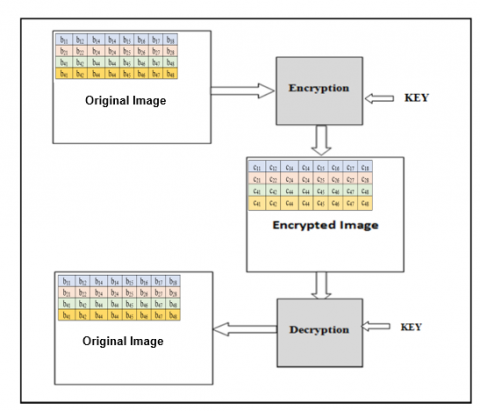Figure 6. Block diagram of image encryption

## 7.png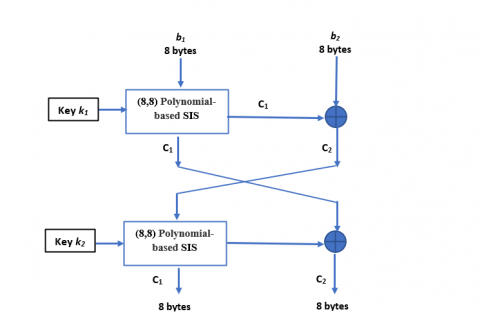Figure 7. The block diagram of the proposed F function

4.4.1 The function F

The core of the proposed F function is (8,8) polynomial-based SIS. For encryption, the input for the F function is two blocks of size 32 bytes pixels constructing 32 polynomials. The output for each polynomial is chained like pipeline and finally produced the encrypted block for the cipher image. The F function steps are described in Algorithm 4:

 Algorithm 4: The proposed F function Input         b1, b2    // two sub blocks each of which is 32 bytes         k1, k2   // two keys each of which is 64 bytes      Output        c1, c2      // two cipher blocks Step 1: Construct (8,8) Polynomial-based SIS for sub block {bj | j=1..8}, the ith cipher value is computed using the following polynomial equation: $c_{j}=\sum_{j=1}^{8} b_{j} k_{i j} \bmod 251$  (11) where i=1...8,  ci is the ith created cipher value for the block b(), kij is the jth key that belongs to the polynomial equation. Therefore, in the case of collecting 8 cipher values (i.e., {ck| k=1...8}), we can retrieve the original values. Step 2: The result from Eq. (6) c1 is XORed with the b2 to produce c2. Step 3: Swap c1 and c2. Step 4: Repeat Step1 for c1. Step 5: The result from step4 c1 is XORed with the c2 to produce c2. Step 6: The output is c1 and c2.
• Example to show the (8,8) Polynomial-based SIS
• Let b be a block from the scrambled image PermROI of size 8 bytes, as shown below.

## 7.1.png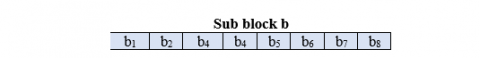• Let k be a secret key block of size 8×8 (i.e., 512 bits).

## 7.2.png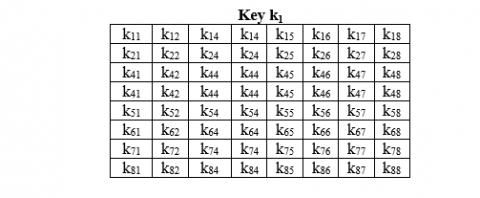• Construct 8×8 (8,8) Polynomial-based SIS for each sub block {bj | j=1..8}, To generate 8 bytes in the encrypted block {cj | j=1..8}, the following polynomials to calculate the encrypted first row in c are as follows:

$c_{1}=b_{1} k_{11}+b_{2} k_{12}+b_{3} k_{14}+b_{4} k_{14}+b_{5} k_{15}+b_{6} k_{16}+$$b_{7} k_{17}+b_{8} k_{18} c_{2}=b_{1} k_{21}+b_{2} k_{22}+b_{3} k_{24}+b_{4} k_{24}+b_{5} k_{25}+b_{6} k_{26}+$$b_{7} k_{27}+b_{8} k_{28}$

$c_{8}=b_{1} k_{81}+b_{2} k_{82}+b_{3} k_{84}+b_{4} k_{84}+b_{5} k_{85}+b_{6} k_{86}+$$b_{7} k_{87}+b_{8} k_{88} ## 7.3.png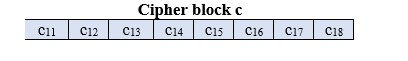Following the diffusion phase, the encrypted image is generated. Clearly, the decryption process is similar to the encryption procedure but in reverse. Figure 9 illustrates examples of encrypted photos, while Figure 10 illustrates the outcome of each stage of the proposed system. ## 8.png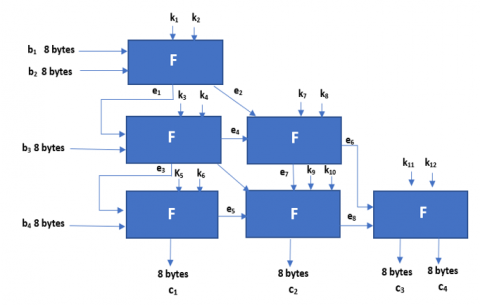Figure 8. The block diagram of the proposed encryption algorithm ## 9.png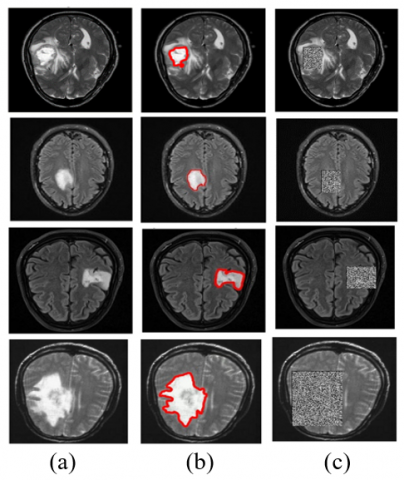Figure 9. The proposed system’s results. Samples of (a) Original image, (b) Detect the ROI, (c) Encrypt the ROI ## 10.png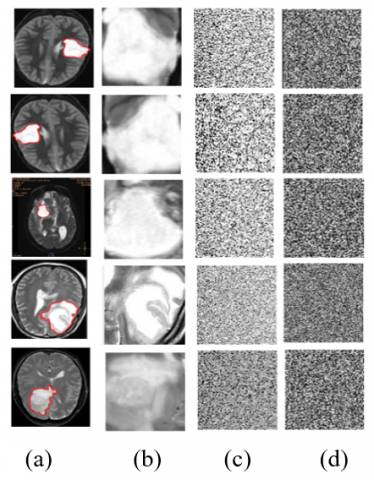Figure 10. The simulation outcomes of the proposed image encryption algorithm steps: (a) Localization of ROI, (b) Extraction of ROI image, (c) Permuting of ROI e; (d) Encrypted image of permuted ROI 5. Experimental Results The suggested system's performance analysis will be validated and implemented using MATLAB 2019b. The medical photos used in this article were obtained from an image database. The following table details the medical images utilized in the simulation and the next section discusses the parameters used to evaluate the proposed work's performance. 5.1 Key space The huge key space is necessary to prevent conducting a thorough search for a key. In other words, solving the issue of selecting the exact key value can be done by trying a range of values until the correct key is found. Additionally, the suggested system makes use of the two independent variables, (x0 and a) and the quadratic map. As a result, the key space is represented by the symbol X0, a . However, the total number of distinct values for (X 0 and a) exceeds the value of 10^{14} because of the fact that (X0 and a) are double-precision numbers. Specifically, the used secret keys are ten initial conditions including X_{1}, X_{1}{ }^{\prime}, X_{2}, X_{2}{ }^{\prime}, X_{3}, X_{3}{ }^{\prime}, X_{4}, X_{4}{ }^{\prime}, X_{5},X_{5}{ }^{\prime}, X_{6}, X_{6}{ }^{\prime}, X_{7}, X_{7}{ }^{\prime}, X_{8}, X_{8}{ }^{\prime}, X_{9}, X_{9}{ }^{\prime}, X_{10} and X_{10}{ }^{\prime} with ten a_{1}, a_{1}^{\prime}, a_{2}, a_{2}^{\prime}, a_{3}, a_{3}^{\prime}, a_{4}, a_{4}^{\prime}, a_{5}, a_{5}^{\prime}, a_{6}, a_{6}^{\prime}, a_{7}, a_{7}^{\prime}, a_{8}$$a_{8}{ }^{\prime}, a_{9}, a_{9}{ }^{\prime}, a_{10}$ and $a_{10}^{\prime}$, where the total number of various values for $X_{0}$  and a is more than $\left(10^{14}\right)^{10} \approx 2^{465}$.  Table 1 shows a comparison of the key space between the algorithm and the other implementations. It is interesting to notice that the used algorithm has a main space greater than that of the majority of the compared algorithms. Because the key space for the enhanced encryption technique is huge in this research, brute force attacks on the suggested algorithm are impracticable.

Table 1. Key space comparison

 ENCRYPTION ALGORITHM KEY SPACE Xiong et al.  $2^{166}$ Sasikaladevi et al.  $2^{131}$ Qiu et al.  $2^{75}$ Liu et al.  $2^{128}$ Proposed algorithm $2^{465}$

5.2 Histogram analysis

The histogram is a graphical representation of the distribution of pixels with varied intensities. In other words, it graphically represents the overall pixel’s numbers with different values of intensities by the (x, y) axis. The histogram of the original and the encrypted images is illustrated in Figure 11. In particular, while the histogram of a totally encrypted image is flat, the selectively encrypted image histogram contains spikes and is identical to the plain image histogram because of the fact that only the pixels are permuted. This result demonstrates that while statistical attacks on the entirely encrypted image are unlikely, they may be possible on the partially encrypted image and histograms accurately show the distribution of pixel values.

An image histogram graphic depicts the frequency of intensity values that appear in an image. Generally, image histograms are used in encryption analyses to evaluate the encryption procedure's performance. In this context, having a consistent histogram assures that the algorithm is capable of withstanding statistical cryptanalysis and our histogram analysis in Figure 11 illustrates a standard uniformity.

## 11.png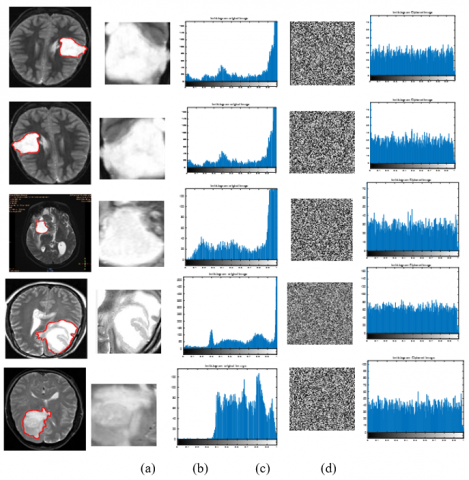Figure 11. (a) Original image, (b) Histogram of the original image, (c) Encrypted image (d) Histogram of the encrypted image

5.3 Analysis of entropy

Entropy is defined as measuring the image's information content, specifically the average number of bits in the image's gray levels. An increase in the value of entropy indicates an increase in the degree of confusion associated with the information conveyed by the image. The information entropy E of discrete 2D images is calculated using Eq. (12) :

$E=-\sum_{i=0}^{n} p_{i} \log _{2}\left(p_{i}\right)$,        (12)

where, pi is a probability of having a gray value when the probability of distributed information is equivalent to the encrypted image. Consequently, the highest entropy is 8 bits, when the probability of each pixel having a value between [0, 255] is 1/256. However, the more confusing information of an image means a higher value of entropy. For the test image in the presented algorithm, Table 2 summarizes all the information entropy for the current algorithm. In this table, the results show that the entropy of the ciphered image data for the samples is nearly close to 8 bits, indicating that the proposed approach has a higher superiority.

Table 2. The entropy of the original and the encrypted images using the proposed algorithm

 IMAGE ORIGINAL IMAGE ENCRYPTION IMAGE Image 1 6.80732 7.9981 Image 2 6.80732 7.9979 Image 3 6.93796 7.9984 Image 4 6.63432 7.9973 Image 5 7.16705 7.9983

The information entropy of an image is an estimation of its unpredictability. Nevertheless, the image's unpredictability is determined by the chance of occurrence of the image's distinct gray levels. The maximum level of randomness is represented by an image with all pixels having the same gray level. Specifically, the randomness of an image indicates its level of confidentiality. Additionally, it signifies information leaking. Entropy also determines the cryptosystem's strength. The formula for computing the information entropy is given below :

$E T(m)=-\sum_{i=0}^{L-1} p\left(m_{i}\right) \times \log _{2}\left(p\left(m_{i}\right)\right)$            (13)

The entropy is denoted as (ET), while the total number of grey level values is represented as L, and p ( $m_{i}$ ) is the probability of a pixel occurrence at each grey level ( $m_{i}$ ) to be considered as random, where a grayscale image with 256 levels should have an entropy of at least 8. An encrypted image with an entropy value near 8 represents a highly random image with minimal leakage, while a result of less than 8 entropy indicates a predictable image that threatens security. This demonstrates the document's consistency. As a result, our algorithm is better in terms of document certainty. Hence, this system is resistant against entropy-based attacks.

Considering the entropy of the original and the encrypted images using the proposed algorithm, the entropy of the original image before encryption ranges from 6.80 to 7.16, and after encryption, it was very close to 8, which is near to the ideal entropy, as shown in the table below.

5.4 NPCR and UACI

An interceptor can make a small change to the original image and observe the resulting ciphered image's modifications. This allows for the observation of the important relationship between the input image and the output image. If significant changes in the ciphered image are noticed in response to a small change in the original image, this indicates that the interceptor can extract the key used for encryption and crack the method .

Two parameters are calculated to determine the algorithm's efficiency, namely NPCR and UACI, which have optimum values of 100 and 33.33, respectively. The number of unified, averaged, and changed intensity and the number of pixels changing at a constant rate is frequently used to assess the plaintext sensitivity and the avalanche effect, as shown in the study  and illustrated in Table 3.

$N P C R=\frac{\sum_{i, j} W(i, j)}{M * N} \times 100$,           (14)

where, $M$ is the width of the image, $N$ is the height of the image, and $W(i, j)$ can be defined as:

$W(i, j)= \begin{cases}1, & \text { if } \operatorname{Cip} 1(i, j) \neq \operatorname{Cip} 2(i, j) \\ 0, & \text { if } \operatorname{Cip} 1(i, j)=\operatorname{Cip} 2(i, j)\end{cases}$

where,

$\operatorname{Cip} 1(i, j)$ is the grey value of the encrypted image and

$\operatorname{Cip} 2(i, j)$ is the grey value of the new encrypted image.

$U A C I \frac{1}{M \times N} \times \sum_{i, j} \frac{a b s(\operatorname{Cip} 1(i, j)-\operatorname{Cip} 2(i, j))}{255} \times 100$          (15)

The results of the above tests for the selected image are shown in Table 3.

Table 3. Encrypted images using the proposed system NPCR and UACI

 Image NPCR UACI Image 1 99.745 40.824 Image 2 99.759 38.660 Image 3 99.804 36.664 Image 4 99.624 35.030 Image 5 99.486 34.784

As can be seen from Table 3, the achieved UACI and NPCR using the proposed system are nearly close to the ideal values, which means that the algorithm is more resistant to different attacks.

5.5 Correlation analysis of adjacent pixels

Correlation coefficients for clearly visible images with proper brightness are equal to one, while for encrypted images, they are significantly reduced values, which are almost equal to zero . A slandered image is closely sampled throughout the grid in all directions. One of the most important objectives of image encryption is to decrease correlations among modified pixels, thereby increasing the apparent degree of protection. In this regard, it is critical to grasp how horizontal, vertical, and diagonal pixels relate to one another. Figure 11 shows maps of the correlation density distribution for test images. Furthermore, Figure 11 illustrates the suggested relative map for the encrypted images by the device. While the image is strongly related with monochromatic images by observing a linear relationship, this association is dramatically reduced when viewing ciphered images. This means that the most advanced image encryption approach is extremely effective and secure. More specifically, the correlation coefficient is denoted by the following equations :

$C_{x y}=\frac{\operatorname{COVR}(x, y)}{\sqrt{V(x)} \sqrt{V(y)}}$        (16)

where, $\operatorname{COVR}(x, y)$  is the covariance between x and y. This is denoted in the study . V (x) is the variable x variance and is given by :

$\operatorname{COVR}(x, y)=\frac{1}{n} \times \sum_{i=1}^{n} E\left[\left(x_{i}-\mu(x)\right)\left(y_{i}-\mu(y)\right)\right]$     (17)

where, x and y are two adjacent pixels’ parameters in the image.

$V(x)=\frac{1}{n} \times \sum_{i=1}^{n}\left[x_{i}-\mu(x)\right]^{2}$     (18)

$\mu(x)$  is the mean of variable x.

$\mu(x)=\frac{1}{n} \times \sum_{i=1}^{n} x_{i}$      (19)

Correlation coefficients were found to be near zero in all directions for the entirely encrypted image. In addition, in the case of the partially encrypted image, the correlation coefficients were between 0 and 1. As a result, this outcome indicates that the algorithm is resistant to geometric attacks. However, the correlation coefficients for the ciphered image and the original image in three different directions are illustrated in Figure 12 and Figure 13.

Greyscale sample images with a size of 256 × 256 are used for the standard digital image ‘Lena’ and compared with our proposed work to verify the security of the proposed image encryption algorithm.

The proposed algorithm, which was applied for the standard image (Lena), is compared with other algorithms by analyzing the entropy, NPCR, UACI, and correlation. The results are shown in Table 4, where the proposed method's histogram and correlation coefficient distributions are good, and the UACI and NPCR results are quite similar to those results obtained with encryption using Polynomial-based Secret Image Sharing and Chaotic Map. Moreover, the value of the information entropy is quite close to the optimal value, which is regarded as a significant achievement of this method. Finally, all the tests were carried out successfully. Thus, it can be said that the proposed method is safe and successful.

## 12.png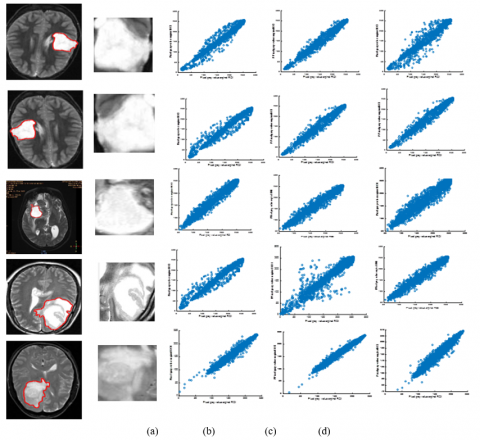Figure 12. Correlation coefficients for the original medical images utilizing the proposed algorithm (a) original image, (b) horizontal, (c) vertical, (d) diagonal correlations

## 13.png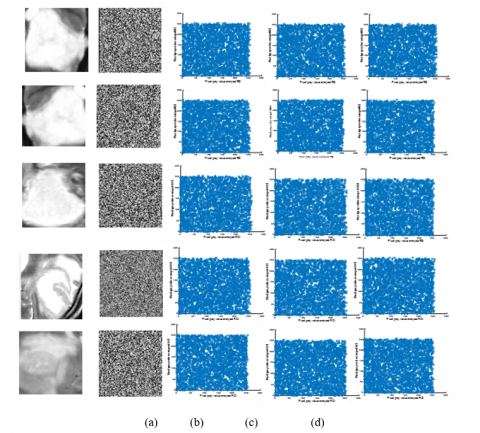Figure 13. Correlation coefficients for the encrypted medical images utilizing the proposed algorithm (a) encrypted image, (b) horizontal, (c) vertical, (d) diagonal correlations

Table 4. Comparison between the proposed algorithm and other methods for ‘Lena’ image

 REFERENCE NPCR UAC I CORRELATION ENTOROPY HORIZONTAL VERTICAL DIAGONAL  99.59 33.42 -0.0028 0.0171 0.0022 7.9891  99.48 33.46 -0.0359 0.0020 0.0019 7.9951  99.39 33.23 / / / 7.9965  99.59 33.52 0.0020 0.0105 0.0019 7.9974  99.51 33.58 0.0023 0.0019 0.0011 7.9975  99.41 33.26 0.0030 0.0024 0.0034 7.9976 Proposed system 99.62 33.57 -0.0016 0.0028 -0.0006 7.9992

5.6 Time complexity

Time complexity describes the amount of time it takes to run an algorithm. It is commonly estimated by counting the number of elementary operations performed by the algorithm, supposing that each elementary operation takes a fixed amount of time to perform. Thus, the amount of time taken and the number of elementary operations performed by the algorithm are taken to differ by at most a constant factor. However, the proposed system aims to reduce the image ciphering time by only ciphering the region of interest rather than the entire image.

Table 5 shows that encryption of entire image needs more time than encryption of region of interest only. So, the execution time is effectively reduced by encrypting only ciphering the region of interest rather than the entire image.

Table 5. The time for encrypting the ROI and the time for encrypting the full image

 Image Size (Original Image) (KB) Size (ROI) (KB) Time of ROI (sec) Time of Encrypt Original Image(sec) Image1 99.5781 3.1094 0.5331 5.8325 Image2 99.4521 2.1357 0.3931 5.3659 Image3 75.7158 10.3203 0.8511 5.1012 Image 4 119.0361 6.7041 0.6341 9.9959 Image5 92.2900 4.2900 0.5555 8.5579
6. Conclusions

Selective image encryption plays an important role in medical image applications as it decreases the computational rate and time. In this paper, an efficient selective image encryption algorithm was designed resulting in a secure and robust encryption scheme to protect medical images based on Polynomial Secret Image Sharing and Chaotic Map. More precisely, the determined region is encrypted to reduce the encryption/decryption time and the image dispensation methods are used to split the image into a region of interest (ROI) and a region of non-interest (RONI). Subsequently, the more important component in ROI is encrypted using a polynomial-based secret image sharing (SIS) and chaotic map system. These techniques produce a cipher of the test image which has good confusion and diffusion features. The experimental result shows that the Polynomial-based SIS and chaotic image encryption are carried out for diffusion and confusion, which are regarded as crucial factors for concealment.

References

 Revanna, C.R., Keshavamurthy, C. (2020). A new partial image encryption method for document images using variance based quad tree decomposition. International Journal of Electrical & Computer Engineering, 10(1): 786-800. https://doi.org/10.11591/ijece.v10i1

 Jang, W., Lee, S.Y. (2020). Partial image encryption using format-preserving encryption in image processing systems for Internet of things environment. International Journal of Distributed Sensor Networks, 16(3): 1550147720914779. https://doi.org/10.1177/1550147720914779

 Ramasamy, P., Ranganathan, V., Kadry, S., Damaševičius, R., Blažauskas, T. (2019). An image encryption scheme based on block scrambling, modified zigzag transformation and key generation using enhanced logistic—Tent map. Entropy, 21(7): 656. https://doi.org/10.3390/e21070656

 Shimal, A.F., Helal, B.H., Hashim, A.T. (2021). Extended of TEA: A 256 bits block cipher algorithm for image encryption. International Journal of Electrical and Computer Engineering, 11(5): 3996-4007.

 Parameshachari, B.D., Panduranga, H.T., Liberata Ullo, S. (2020). Analysis and computation of encryption technique to enhance security of medical images. In IOP Conference Series: Materials Science and Engineering, 925(1): 012028. https://doi.org/10.1088/1757-899X/925/1/012028

 Hamad, A., Farhan, A.K. (2020). Image encryption algorithm based on substitution principle and shuffling scheme. Engineering and Technology Journal, 38(3): 98-103. https://doi.org/10.30684/etj.v38i3B.433

 Yuan, L., Ebrahimi, T. (2017). Image privacy protection with secure JPEG transmorphing. IET Signal Processing, 11(9): 1031-1038. https://doi.org/10.1049/iet-spr.2016.0756

 Das, S., Kundu, M.K. (2013). Effective management of medical information through ROI-lossless fragile image watermarking technique. Computer Methods and Programs in Biomedicine, 111(3): 662-675. https://doi.org/10.1016/j.cmpb.2013.05.027

 Goel, A., Chaudhari, K. (2016). Median based pixel selection for partial image encryption. In 2016 Sixth International Conference on Image Processing Theory, Tools and Applications (IPTA), pp. 1-5. https://doi.org/10.1109/IPTA.2016.7820931

 Belazi, A., Abd El-Latif, A.A., Diaconu, A.V., Rhouma, R., Belghith, S. (2017). Chaos-based partial image encryption scheme based on linear fractional and lifting wavelet transforms. Optics and Lasers in Engineering, 88: 37-50. https://doi.org/10.1016/j.optlaseng.2016.07.010

 Som, S., Palit, S., Dey, K. (2017). Evaluating the performance of a chaos based partial image encryption scheme. In Advanced Computing and Systems for Security, 173-185. https://doi.org/10.1007/978-981-10-3409-1_12

 Ismail, S.M., Said, L.A., Radwan, A.G., Madian, A.H., Abu-Elyazeed, M.F. (2018). Generalized double-humped logistic map-based medical image encryption. Journal of Advanced Research, 10: 85-98. https://doi.org/10.1016/j.jare.2018.01.009

 Sankaradass, V., Murali, P., Tholkapiyan, M. (2018). Region of Interest (ROI) based image encryption with sine map and Lorenz system. In International Conference on ISMAC in Computational Vision and Bio-Engineering, pp. 493-502. https://doi.org/10.1007/978-3-030-00665-5_49

 Darwish, S.M. (2019). A modified image selective encryption-compression technique based on 3D chaotic maps and arithmetic coding. Multimedia Tools and Applications, 78(14): 19229-19252. https://doi.org/10.1007/s11042-019-7256-6

 Zhou, J., Li, J., Di, X. (2020). A novel lossless medical image encryption scheme based on game theory with optimized ROI parameters and hidden ROI position. IEEE Access, 8: 122210-122228. https://doi.org/10.1109/ACCESS.2020.3007550

 Khashan, O.A., AlShaikh, M. (2020). Edge-based lightweight selective encryption scheme for digital medical images. Multimedia Tools and Applications, 79(35): 26369-26388. https://doi.org/10.1007/s11042-020-09264-z

 Cun, Q., Tong, X., Wang, Z., Zhang, M. (2021). Selective image encryption method based on dynamic DNA coding and new chaotic map. Optik, 243: 167286. https://doi.org/10.1016/j.ijleo.2021.167286

 Wen, H., Zhang, C., Chen, P., Chen, R., Xu, J., Liao, Y., Ke, J. (2021). A quantum chaotic image cryptosystem and its application in IoT secure communication. IEEE Access, 9: 20481-20492. https://doi.org/10.1109/ACCESS.2021.3054952

 Pandurangi Ramacharya, B., Patil, M.R., Keralkar, S. (2022). Fast partial image encryption with fuzzy logic and chaotic mapping. Evolutionary Intelligence, 1-17. https://doi.org/10.1007/s12065-021-00693-9

 Wu, K.S. (2013). A secret image sharing scheme for light images. EURASIP Journal on Advances in Signal Processing, 2013(1): 1-5. https://doi.org/10.1186/1687-6180-2014-49

 Shamir, A. (1979). How to share a secret. Communications of the ACM, 22(11): 612-613. https://doi.org/10.1145/359168.359176

 Dutta, R., Annappa, B. (2014). Protection of data in unsecured public cloud environment with open, vulnerable networks using threshold-based secret sharing. Netw. Protoc. Algorithms, 6(1): 58-75.

 Mohammed, R.S., Sadkhan, S.B. (2016). Speech scrambler based on proposed random chaotic maps. In 2016 Al-Sadeq International Conference on Multidisciplinary in IT and Communication Science and Applications (AIC-MITCSA), pp. 1-6. https://doi.org/10.1109/AIC-MITCSA.2016.7759928

 Salman, L.A., Hashim, A.T., Hasan, A.M. (2022). Automated brain tumor detection of MRI image based on hybrid image processing techniques. TELKOMNIKA (Telecommunication Computing Electronics and Control), 20(4). http://doi.org/10.12928/telkomnika.v20i4.22760

 Rehman, A.U., Wang, H., Shahid, M.M.A., Iqbal, S., Abbas, Z., Firdous, A. (2019). A selective cross-substitution technique for encrypting color images using chaos, DNA rules and SHA-512. IEEE Access, 7: 162786-162802. https://doi.org/10.1109/ACCESS.2019.2951749

 Hashim, A.T., Jassem, A.H., Ali, S.A. (2021). A novel design of blowfish algorithm for image security. In Journal of Physics: Conference Series, 1818(1): 012085. https://doi.org/10.1088/1742-6596/1818/1/012085

 Hashim, A.T., Jabbar, A.K., Hassan, Q.F. (2021). Medical image encryption based on hybrid AES with chaotic map. Journal of Physics: Conference Series 1973(1): 012037. https://doi.org/10.1088/1742-6596/1973/1/012037

 Xiong, Z., Wu, Y., Ye, C., Zhang, X., Xu, F. (2019). Color image chaos encryption algorithm combining CRC and nine palace map. Multimedia Tools and Applications, 78(22): 31035-31055. https://doi.org/10.1007/s11042-018-7081-3

 Sasikaladevi, N., Geetha, K., Sriharshini, K., Durga Aruna, M. (2019). RADIANT-hybrid multilayered chaotic image encryption system for color images. Multimedia Tools and Applications, 78(9): 11675-11700. https://doi.org/10.1007/s11042-018-6711-0

 Qiu, W.C., Yan, S.J. (2019). An image encryption algorithm based on the combination of low - dimensional chaos and high - dimensional chaos. 2019 3rd International Conference on Electronic Information Technology and Computer Engineering (EITCE), pp. 684-687. https://doi.org/10.1109/EITCE47263.2019.9094882

 Liu, H., Jin, C. (2017). A novel color image encryption algorithm based on quantum chaos sequence. 3D Research, 8(1): 1-13.

 Abd Aljabar, R.W., Hassanb, N.F. (2021). Encryption VOIP based on generated biometric key for RC4 algorithm. Engineering and Technology Journal, 39(1B): 209-221. https://doi.org/10.30684/etj.v39i1B.1755

 Jirjees, S.W., Nasser, A.R., Mahmood, A.M. (2021). RoundPIN: Shoulder surfing resistance for PIN entry with randomize keypad. International Journal of Safety and Security Engineering, 11(6): 697-702. https://doi.org/10.18280/ijsse.110610

 Chen, X., Hu, C.J. (2017). Adaptive medical image encryption algorithm based on multiple chaotic mapping. Saudi Journal of Biological Sciences, 24(8): 1821-1827. https://doi.org/10.1016/j.sjbs.2017.11.023

 Zhou, N., Pan, S., Cheng, S., Zhou, Z. (2016). Image compression–encryption scheme based on hyper-chaotic system and 2D compressive sensing. Optics & Laser Technology, 82: 121-133. https://doi.org/10.1016/j.optlastec.2016.02.018

 Natiq, H., Al-Saidi, N.M.G., Said, M.R.M., Kilicman, A. (2018). A new hyperchaotic map and its application for image encryption. The European Physical Journal Plus, 133(1): 1-14. https://doi.org/10.1140/epjp/i2018-11834-2

 Liu, L., Hao, S., Lin, J., Wang, Z., Hu, X., Miao, S. (2018). Image block encryption algorithm based on chaotic maps. IET Signal Processing, 12(1): 22-30. https://doi.org/10.1049/iet-spr.2016.0584

 Enayatifar, R., Abdullah, A.H., Isnin, I.F., Altameem, A., Lee, M. (2017). Image encryption using a synchronous permutation-diffusion technique. Optics and Lasers in Engineering, 90: 146-154. https://doi.org/10.1016/j.optlaseng.2016.10.006

 Liu, W., Sun, K., Zhu, C. (2016). A fast image encryption algorithm based on chaotic map. Optics and Lasers in Engineering, 84: 26-36. https://doi.org/10.1016/j.optlaseng.2016.03.019